## Section Exercises

1. Discuss the meaning of a sequence. If a finite sequence is defined by a formula, what is its domain? What about an infinite sequence?

2. Describe three ways that a sequence can be defined.

3. Is the ordered set of even numbers an infinite sequence? What about the ordered set of odd numbers? Explain why or why not.

4. What happens to the terms ${a}_{n}$ of a sequence when there is a negative factor in the formula that is raised to a power that includes $n?$ What is the term used to describe this phenomenon?

5. What is a factorial, and how is it denoted? Use an example to illustrate how factorial notation can be beneficial.

For the following exercises, write the first four terms of the sequence.

6. ${a}_{n}={2}^{n}-2$

7. ${a}_{n}=-\frac{16}{n+1}$

8. ${a}_{n}=-{\left(-5\right)}^{n - 1}$

9. ${a}_{n}=\frac{{2}^{n}}{{n}^{3}}$

10. ${a}_{n}=\frac{2n+1}{{n}^{3}}$

11. ${a}_{n}=1.25\cdot {\left(-4\right)}^{n - 1}$

12. ${a}_{n}=-4\cdot {\left(-6\right)}^{n - 1}$

13. ${a}_{n}=\frac{{n}^{2}}{2n+1}$

14. ${a}_{n}={\left(-10\right)}^{n}+1$

15. ${a}_{n}=-\left(\frac{4\cdot {\left(-5\right)}^{n - 1}}{5}\right)$

For the following exercises, write the first eight terms of the piecewise sequence.

16. ${a}_{n}=\begin{cases}\left(−2\right)^{n}−2 \hfill& \text{if }n\text{ is even} \\ \left(2\right)^{n−1} \hfill& \text{if }n\text{ is odd}\end{cases}$

17. ${a}_{n}=\begin{cases}\frac{n^{2}}{2n+1}\hfill& \text{if }n\le5 \\ n^{2}−5 \hfill& \text{if }n>5\end{cases}$

18. ${a}_{n}=\begin{cases}\left(2n+1\right)^{2}\hfill& \text{if }n\text{ is divisible by }4 \\ \frac{2}{n}\hfill& \text{if }n\text{ is not divisible by }4\end{cases}$

19. ${a}_{n}=\begin{cases}−0.6\times5^{n−1}\hfill& \text{if }n\text{ is prime or }1 \\ 2.5\times\left(−2\right)^{n−1}\hfill& \text{if }n\text{ is composite}\end{cases}$

20. ${a}_{n}=\begin{cases}4\left({n}^{2}-2\right)&\text{ if }{ n }\le{3}\text{ or }{ n }>{ 6 }\\\frac{{n}^{2}-2}{4} & \text{ if }{ 3 }<{ n }\le{ 6 }\end{cases}$

For the following exercises, write an explicit formula for each sequence.

21. $4, 7, 12, 19, 28,\dots$

22. $-4,2,-10,14,-34,\dots$

23. $1,1,\frac{4}{3},2,\frac{16}{5},\dots$

24. $0,\frac{1-{e}^{1}}{1+{e}^{2}},\frac{1-{e}^{2}}{1+{e}^{3}},\frac{1-{e}^{3}}{1+{e}^{4}},\frac{1-{e}^{4}}{1+{e}^{5}},\dots$

25. $1,-\frac{1}{2},\frac{1}{4},-\frac{1}{8},\frac{1}{16},\dots$

For the following exercises, write the first five terms of the sequence.

26. ${a}_{1}=9,\text{ }{a}_{n}={a}_{n - 1}+n$

27. ${a}_{1}=3,\text{ }{a}_{n}=\left(-3\right){a}_{n - 1}$

28. ${a}_{1}=-4,\text{ }{a}_{n}=\frac{{a}_{n - 1}+2n}{{a}_{n - 1}-1}$

29. ${a}_{1}=-1,\text{ }{a}_{n}=\frac{{\left(-3\right)}^{n - 1}}{{a}_{n - 1}-2}$

30. ${a}_{1}=-30,\text{ }{a}_{n}=\left(2+{a}_{n - 1}\right){\left(\frac{1}{2}\right)}^{n}$

For the following exercises, write the first eight terms of the sequence.

31. ${a}_{1}=\frac{1}{24},{\text{ a}}_{2}=1,\text{ }{a}_{n}=\left(2{a}_{n - 2}\right)\left(3{a}_{n - 1}\right)$

32. ${a}_{1}=-1,{\text{ a}}_{2}=5,\text{ }{a}_{n}={a}_{n - 2}\left(3-{a}_{n - 1}\right)$

33. ${a}_{1}=2,{\text{ a}}_{2}=10,\text{ }{a}_{n}=\frac{2\left({a}_{n - 1}+2\right)}{{a}_{n - 2}}$

For the following exercises, write a recursive formula for each sequence.

34. $-2.5,-5,-10,-20,-40,\dots$

35. $-8,-6,-3,1,6,\dots$

36. $2,\text{ }4,\text{ }12,\text{ }48,\text{ }240,\text{ }\dots$

37. $35,\text{ }38,\text{ }41,\text{ }44,\text{ }47,\text{ }\dots$

38. $15,3,\frac{3}{5},\frac{3}{25},\frac{3}{125},\cdots$

For the following exercises, evaluate the factorial.

39. $6!$

40. $\left(\frac{12}{6}\right)!$

41. $\frac{12!}{6!}$

42. $\frac{100!}{99!}$

For the following exercises, write the first four terms of the sequence.

43. ${a}_{n}=\frac{n!}{{n}^{\text{2}}}$

44. ${a}_{n}=\frac{3\cdot n!}{4\cdot n!}$

45. ${a}_{n}=\frac{n!}{{n}^{2}-n - 1}$

46. ${a}_{n}=\frac{100\cdot n}{n\left(n - 1\right)!}$

For the following exercises, graph the first five terms of the indicated sequence

47. ${a}_{n}=\frac{{\left(-1\right)}^{n}}{n}+n$

48. ${a}_{n}=\begin{cases}\frac{4+n}{2n} & \text{if }n\text{ is even} \\ 3+n & \text{if }n\text{ is odd}\end{cases}$

49. ${a}_{1}=2,\text{ }{a}_{n}={\left(-{a}_{n - 1}+1\right)}^{2}$

50. ${a}_{n}=1,\text{ }{a}_{n}={a}_{n - 1}+8$

51. ${a}_{n}=\frac{\left(n+1\right)!}{\left(n - 1\right)!}$

For the following exercises, write an explicit formula for the sequence using the first five points shown on the graph.

52.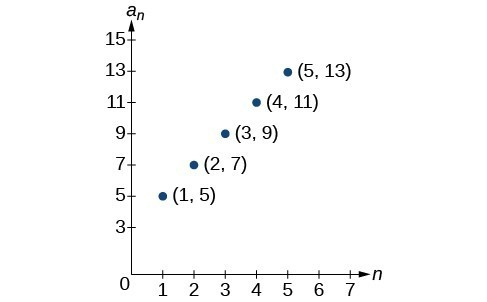53.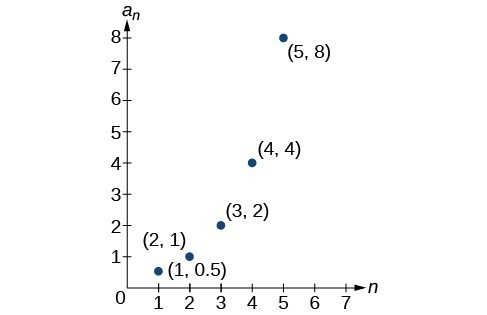54.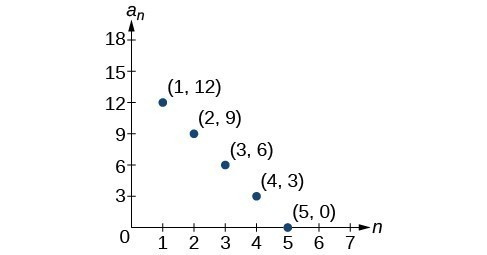For the following exercises, write a recursive formula for the sequence using the first five points shown on the graph.

55.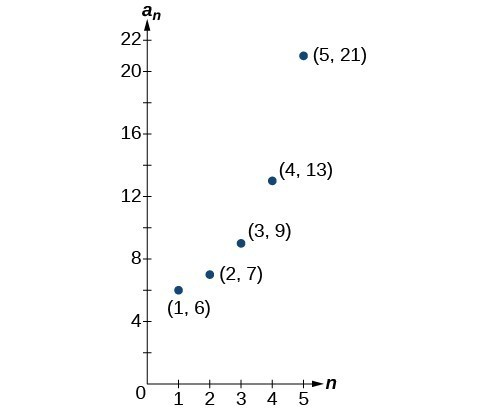56.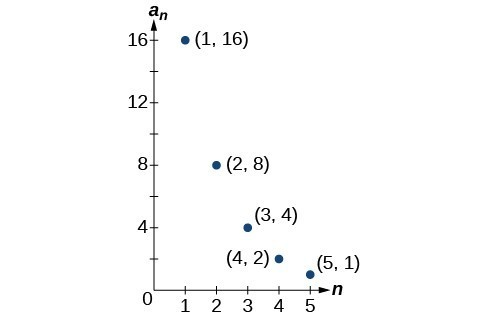Follow these steps to evaluate a sequence defined recursively using a graphing calculator:

• On the home screen, key in the value for the initial term ${a}_{1}$ and press [ENTER].
• Enter the recursive formula by keying in all numerical values given in the formula, along with the key strokes [2ND] ANS for the previous term ${a}_{n - 1}$. Press [ENTER].
• Continue pressing [ENTER] to calculate the values for each successive term.

For the following exercises, use the steps above to find the indicated term or terms for the sequence.

57. Find the first five terms of the sequence ${a}_{1}=\frac{87}{111},\text{ }{a}_{n}=\frac{4}{3}{a}_{n - 1}+\frac{12}{37}$. Use the >Frac feature to give fractional results.

58. Find the 15th term of the sequence ${a}_{1}=625, {a}_{n}=0.8{a}_{n - 1}+18$.

59. Find the first five terms of the sequence ${a}_{1}=2, {a}_{n}={2}^{\left[\left({a}_{n}-1\right)-1\right]}+1$.

60. Find the first ten terms of the sequence ${a}_{1}=8,\text{ }{a}_{n}=\frac{\left({a}_{n - 1}+1\right)!}{{a}_{n - 1}!}$.

61. Find the tenth term of the sequence ${a}_{1}=2,\text{ }{a}_{n}=n{a}_{n - 1}$

Follow these steps to evaluate a finite sequence defined by an explicit formula. Using a TI-84, do the following.

• In the home screen, press [2ND] LIST.
• Scroll over to OPS and choose “seq(“ from the dropdown list. Press [ENTER].
• In the line headed “Expr:” type in the explicit formula, using the $\left[\text{X,T},\theta ,n\right]$ button for $n$
• In the line headed “Variable:” type in the variable used on the previous step.
• In the line headed “start:” key in the value of $n$ that begins the sequence.
• In the line headed “end:” key in the value of $n$ that ends the sequence.
• Press [ENTER] 3 times to return to the home screen. You will see the sequence syntax on the screen. Press [ENTER] to see the list of terms for the finite sequence defined. Use the right arrow key to scroll through the list of terms.

Using a TI-83, do the following.

• In the home screen, press [2ND] LIST.
• Scroll over to OPS and choose “seq(“ from the dropdown list. Press [ENTER].
• Enter the items in the order “Expr”, “Variable”, “start”, “end” separated by commas. See the instructions above for the description of each item.
• Press [ENTER] to see the list of terms for the finite sequence defined. Use the right arrow key to scroll through the list of terms.

For the following exercises, use the steps above to find the indicated terms for the sequence. Round to the nearest thousandth when necessary.

62. List the first five terms of the sequence ${a}_{n}=-\frac{28}{9}n+\frac{5}{3}$.

63. List the first six terms of the sequence ${a}_{n}=\frac{{n}^{3}-3.5{n}^{2}+ 4.1n - 1.5}{2.4n}$.

64. List the first five terms of the sequence ${a}_{n}=\frac{15n\cdot {\left(-2\right)}^{n - 1}}{47}$

65. List the first four terms of the sequence ${a}_{n}={5.7}^{n}+0.275\left(n - 1\right)!$

66. List the first six terms of the sequence ${a}_{n}=\frac{n!}{n}$.

67. Consider the sequence defined by ${a}_{n}=-6 - 8n$. Is ${a}_{n}=-421$ a term in the sequence? Verify the result.

68. What term in the sequence ${a}_{n}=\frac{{n}^{2}+4n+4}{2\left(n+2\right)}$ has the value $41?$ Verify the result.

69. Find a recursive formula for the sequence $1,\text{ }0,\text{ }-1,\text{ }-1,\text{ }0,\text{ }1,\text{ }1,\text{ }0,\text{ }-1,\text{ }-1,\text{ }0,\text{ }1,\text{ }1,\text{ }…\text{ }$. (Hint: find a pattern for ${a}_{n}$ based on the first two terms.)

70. Calculate the first eight terms of the sequences ${a}_{n}=\frac{\left(n+2\right)!}{\left(n - 1\right)!}$ and ${b}_{n}={n}^{3}+3{n}^{2}+2n$, and then make a conjecture about the relationship between these two sequences.

71. Prove the conjecture made in the preceding exercise.